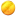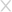• 手机版微信扫一扫

• 投稿

## 一个FreeBSD下的通信协议监控程序

2018-08-05+12 158352人围观 ，发现 2 个不明物体

BLOG

## 实现

### 1. 替换通信协议的处理函数

ICMP

``````{
.pr_type =		SOCK_RAW,
.pr_domain =		&inetdomain,
.pr_protocol =		IPPROTO_ICMP,
.pr_input =		icmp_input,
.pr_ctloutput =		rip_ctloutput,
.pr_usrreqs =		&rip_usrreqs
},
``````

TCP

``````{
.pr_type =		SOCK_STREAM,
.pr_domain =		&inetdomain,
.pr_protocol =		IPPROTO_TCP,
.pr_flags =		PR_CONNREQUIRED|PR_IMPLOPCL|PR_WANTRCVD,
.pr_input =		tcp_input,
.pr_ctlinput =		tcp_ctlinput,
.pr_ctloutput =		tcp_ctloutput,
.pr_init =		tcp_init,
.pr_slowtimo =		tcp_slowtimo,
.pr_drain =		tcp_drain,
.pr_usrreqs =		&tcp_usrreqs
},
``````

UDP

``````{
.pr_type = 		SOCK_DGRAM,
.pr_domain = 		&inetdomain,
.pr_protocol = 		IPPROTO_UDP,
.pr_input = 		udp_input,
.pr_ctlinput = 		udp_ctlinput,
.pr_ctloutput = 	ip_ctloutput,
.pr_init = 		udp_init,
.pr_usrreqs = 		&udp_usrreqs
},``````

``````case MOD_LOAD:
/* Replace udp_input with udp_input_hook. */
inetsw[ip_protox[IPPROTO_UDP]].pr_input = udp_input_hook;
break;
``````

### 2. 设计hook函数

``````int
udp_input_hook(struct mbuf **mp, int *offp, int proto);``````

``````if (iphlen > sizeof (struct ip)) {
ip_stripoptions(m);
iphlen = sizeof(struct ip);
}
``````

``````ip = mtod(m, struct ip *);
if (m->m_len < iphlen + sizeof(struct udphdr)) {
if ((m = m_pullup(m, iphlen + sizeof(struct udphdr))) == NULL) {

UDPSTAT_INC(udps_hdrops);
return (IPPROTO_DONE);
}
ip = mtod(m, struct ip *);
}
``````

``````uh = (struct udphdr *)((caddr_t)ip + iphlen);
``````

``````m->m_len -= iphlen + sizeof(struct udphdr);
m->m_data += iphlen + sizeof(struct udphdr);
``````

``printf("load=%s \n", m->m_data);``

``````m->m_len += iphlen + sizeof(struct udphdr);
m->m_data -= iphlen + sizeof(struct udphdr);
``````

``return udp_input(mp, offp, proto);``

## 后记

*本文作者：rochek，转载请注明来自FreeBuf.COM### 已有 2 条评论

•test  2018-08-10 回复 1楼

请教一下，您的项目选择freebsd作为平台的主要出发点（或者优势）都有哪些？

• (2级) 猫奴  2018-08-11 回复

@ test BSD协议更宽松。
不用这么正式，只是个写着有趣的小项目而已。### 活动预告### 填写个人信息

• 可以给我们打个分吗？